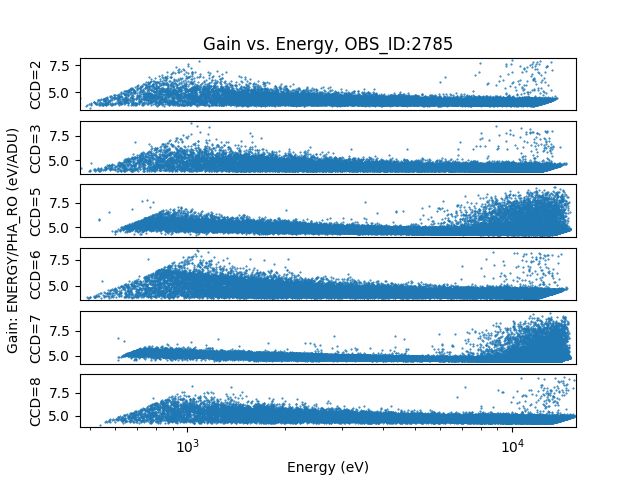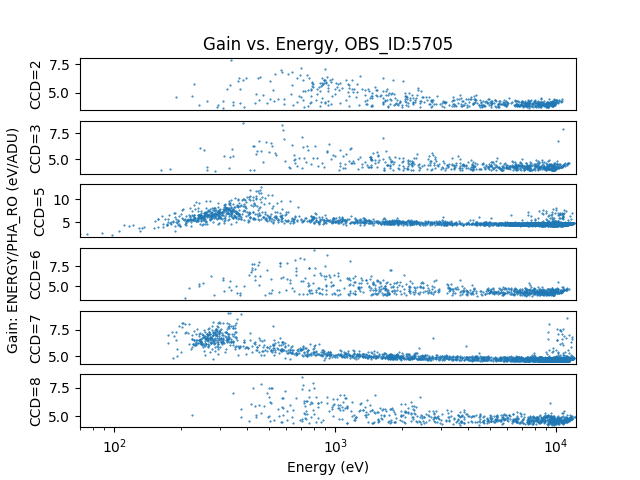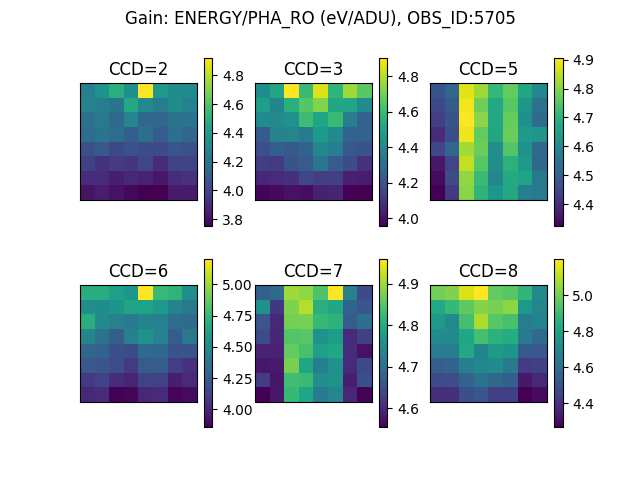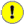## Understanding ACIS on-orbit energy filters

### Introduction

Q: What ENERGY range was used with my ACIS observation?

This is a deceptively complicated question. All ACIS observations have some energy filter applied on-orbit. The default PHA filter allows events with energies in approximately the 0.2:14 keV range.

These energy limits are at or beyond the sensitivity range of the instrument and users typically exclude data approaching these boundaries.

However, observers can specify an arbitrary non-standard energy filter to be used on-orbit. The only advantage to doing so is to avoid telemetry saturation; nevertheless observers have requested them for many observations with modest count-rates.

Given the arbitrary nature of these limits, it may impact users' analysis. For example, typically when scaling the ACIS blank sky background files users will use the data in 9-12keV range; however, if a high energy filter has been applied on-orbit then data in this range may be missing leading to an incorrect scaling.

### From Observer to Observation: How are filters implemented?

When observers request an arbitrary energy filter they supply an energy range in units of keV.

The ACIS instrument team converts those values into Pulse Height Amplitudes, PHA, in units of ADU using a gain formula appropriate for the CCD where the target is located.

A PHA value is computed for each event by the on-board computer, and only those events for which this value is within the specified PHA range are sent to the ground.

The PHA filter used on-orbit is stored in the ACIS Level 0 Window File (win0.fits) and the Level 1 mask file (msk1.fits). In the latter file, the values may be found in the PHAMIN and PHAMAX columns.ChaSeR

The Chandra Observation Catalog (OCAT) stores the energy range the observer proposed (if any). During the observation planning phase, that energy range may be altered or eliminated. ChaSeR returns the values stored in the OCAT. The mask file is the definitive source of what filter was actually used.

#### Example Datasets

Consider the following two observations: OBS_ID=2785 and OBS_ID=5705.

According to Details section in ChaSeR, the observer for OBS_ID=2785 requested a low enegy Event filter with

```    Lower Threshold: 	0.5 keV 		Energy Range: 	12.0 keV
```

and for OBS_ID=5705 the observer requested a high energy event filter with

```    Lower Threshold: 	0.1 keV 		Energy Range: 	9.9 keV
```

The mask file, msk1.fits, contains the actual PHA filters. The data for each CCD are stored in separate blocks (FITS extensions). For OBS_ID 2785, CCD_ID=7, the mask file has these ranges:

```unix% dmlist 2785/secondary/acisf02785_000N003_msk1.fits.gz"[mask7][cols phamin,phamax]" data,clean
#  PHAMIN PHAMAX
126       3124
126       3124
126       3124
```

and for OBS_ID 5705, CCD_ID=7, the ranges in the mask file are

```unix% dmlist 5705/secondary/acisf05705_000N003_msk1.fits.gz"[mask7][cols phamin,phamax]" data,clean
#  PHAMIN PHAMAX
26       2499
26       2499
26       2499
```Why are there multiple rows in the mask file?

There are 3 rows in the mask as a result of the logic needed to capture the fact that the outer 1-pixel border around each CCD is inactive. However, it is worth noting that any|every spatial window can have a different energy filter applied.

## Analyzing Datasets with on-orbit energy filters

The complication with analyzing observations using non-standard on-orbit energy filters is that the events filtered on-orbit are based on the PHA value computed on-orbit. This value is referred to as: PHA_RO (PHA Read Out). The PHAMIN and PHAMAX values in the mask file are PHA_RO.

The PHA value computed by acis_process_events includes additional calibrations such as temperature dependent CTI corrections. In addition, the gain conversion from PHA to ENERGY includes additional ground-only calibrations : spatially varying non-linear gain maps and time dependent gain adjustments.

To express the simple PHAMIN:PHAMAX (PHA_RO) range stored in the mask file in ENERGY units is then very complicated. The combined gain varies non-linearly with energy, varies spatially across each CCD, and has short-term time dependencies (temperature) and long-term time dependencies.ACIS Energy Calibration Crib Sheet

These relationships apply to observations done with DATAMODE=FAINT, VFAINT, or CC33_FAINT, (ie not GRADED mode):

PHAS are the 3x3|5x5 event island pulse height values.

PHA is the sum of the good pixels in the island.

Level 1 PHAS are the Level 0 PHAS values with the bias subtracted and overclock corrected.

Remember: The ENERGY value here is the center of the statistical distribution of true energies corresponding to that PHA value. The PHA is related to true energy via an RMF.

The PHAS_ADJ values are not stored in the standard event file.

## Visualize gain variation: Part 1: vs. energy

One way to visualize this complicated mapping from PHA_RO to ENERGY is to simply plot the values for each event in the event file.

The results are shown for the two datasets

### Figure 1: Gain vs. Energy for OBS_ID 2785### Figure 1: Gain vs. Energy for OBS_ID 2785

The gain (simply defined as ENERGY divided by PHA_RO) is shown for each CCD and plotted vs. ENERGY

The plot was created using this Python script.

There are three important features to notice. First that the plots show a lot of scatter; the gain is not constant vs. energy. Second, the gain is non-linear, especially at low energies. Thirdly, the relationship differs markedly from chip to chip.

### Figure 2: Gain vs. Energy for OBS_ID 5705### Figure 2: Gain vs. Energy for OBS_ID 5705

Same as Figure 1; but for OBS_ID 5705. Note: There are fewer events (plots are less dense) and the X-axis covers a different energy range.

A closer inspection, not shown here, would reveal that each of each per-CCD subplot has 4 separate curves with their own scatter. These are for each of the 4 readout nodes. The nominal gain is set separately for each amplifier attached to each node.

## Visualize gain variation: Part 2: vs. detector location

To understand the nature of the scatter, we can look at how the gain varies spatially.

If we ignore the non-linearities in the gain, one way to visualize the spatial dependency is to create an approximate gain map in chip coordinates from the event data. To do this the event data for each chip are binned in chip coordinates using a large blocking factor (so that each "pixel" has a statistically significant number of events); the bins are weighted by the sum of the event ENERGY values and separately the sum of the events PHA_RO values. The approximate gain map is then simply the total ENERGY map divided by the total PHA_RO map.

The basic ciao commands then look something like:

```unix% dmcopy "evt.fits[ccd_id=1][bin chip=::64;energy]" energy.img
unix% dmcopy "evt.fits[ccd_id=1][bin chip=::64;pha_ro]" pha_ro.img
unix% dmimgcalc energy.img pha_ro.img gain.img div
```

which is repeated for each CCD. The blocking factor (eg 64 in the example above) may need to be adjusted to obtain enough events in each pixel. The gain maps are shown below.

### Figure 3: Gain Map for OBS_ID 2785### Figure 3: Gain Map for OBS_ID 2785

The gain (simply defined as ENERGY divided by PHA_RO) is shown for each CCD. Each image is in chip coordinates. Bottom left corner is (chipx,chipy)=(1,1). Each pixel represents the mean gain in a 64x64 pixel region.

The plot was created using this Python script.

There are a few important features to notice. First is the gradient in the vertical (chipy) direction. This is the result of the CTI corrections when going from PHAS to PHAS_ADJ. The second feature is the difference between the 4 nodes on each chip, dividing each chip into 4 vertical stripes.Each CCD is readout through 4 amplifiers; the gain is set separately for each. Thirdly, as shown before, the relationship differs markedly from chip to chip.

### Figure 4: Gain Map for OBS_ID 5705### Figure 4: Gain Map for OBS_ID 5705

Same as Figure 3 for OBS_ID 5705; but the blocking factor has been increased to 128. Due to fewer events, a larger blocking factor was needed to fully populate the images.

## Okay, it's complicated. Is there a simple answer?

Generally what users want to know when they ask the question: What ENERGY range was used with my ACIS observation? is really:

Above what minimum energy do I have all the events?
Below what maximum energy do I have all the events?

The approximate gain maps created above can be used to calculate a conservative estimate of these limits.

If users simply energy filter the event file on the observer-specified energy range, they will actually be including a different and unknown fraction of the events in that energy range in different areas due to the gain variations. Therefore, it is preferable to filter on a more restricted energy range where users can expect to have 100% of the events are passing the on-board filter.

To compute a conservative, approximate lower energy threshold for each chip we can find the maximum gain in the gain map and apply it to the PHAMIN value from the mask file.

Similarly to compute a conservative, approximate upper energy threshold for each chip we can find the minimum gain and apply it to the PHAMAX.

Conceptually, it is just running

```unix% dmstat gain.img centroid=no
```

and using the min and max values to scale the PHAMIN and PHAMAX values in the mask file.

Using a modified version of the gain map plotting script we can compute these approximate limits.

For OBS_ID 2785 we get the following results per CCD:

```#CCD    PHAMIN  PHAMAX  G_min   G_max   E_min   E_max
2       126     3124    3.8     4.4     559.4   11720.0
3       126     3124    3.9     4.8     602.8   12294.4
5       126     3124    4.3     4.9     617.6   13547.9
6       126     3124    3.9     5.0     630.4   12033.2
7       126     3124    4.5     5.0     625.8   14123.8
8       126     3124    4.2     5.2     652.7   13248.6
```

Where G stands for gain and E for ENERGY. The PHAMIN and PHAMAX values are from the mask file.

Remember the values in ChaSeR were 0.5:12. Then for a conservative limit for all chips we take the maximum, minimum energy; suggesting that all events with energy ~0.65 keV were telemetered.

For OBSID 5705 we get

```#CCD    PHAMIN  PHAMAX  G_min   G_max   E_min   E_max
2       39      2499    3.8     4.9     192.1   9383.1
3       39      2499    4.0     4.9     191.3   9892.4
5       26      2499    4.3     4.9     127.6   10808.6
6       39      2499    3.9     5.2     202.8   9642.1
7       26      2499    4.6     5.0     128.9   11380.3
8       39      2499    4.3     5.2     202.8   10662.5
```

The values in ChaSeR are 0.1:9.9. The upper energy limit computed this way suggests that all events on all chips with energies less than 9.3 keV were telemetered. The proposed lower threshold, 0.1keV, was not implemented and the standard PHAMIN values were used instead.Important

Remember that these values are just approximations computed by ignoring the nonlinearity in the gain.

## Summary

Knowing the energy boundaries of a dataset is essential when performing certain types of analysis, such as when trying to scale the blank sky background files.

This document has described how complicated it is to establish robust energy boundaries when on-orbit energy filters are used.

Users can compute a conservative estimate of the energy range by using the event data to compute approximate gain maps and applying them to the limits stored in the Level 1 mask file, msk1.fits.

The Chandra Data Archive has a heterogeneous mix of datasets with arbitrary energy filters applied. Many of these filters are only minor variations to the default; however, some filters are extreme. For example, OBS_ID 2279 (and the related sequence of Galactic Center mosaic) has a lower energy limit ~1.0 keV. There are also observations such as OBS_ID 13175 (and the rest of the grid used for this comet observation) which use a high energy limit of only ~2.0keV. Beyond just background scaling, attempting to do spectral fitting with these datasets may be problematic.Final Note: bias calibration

There is a bias image (for each CCD) used on-orbit to compute the PHA value which is then used to filter events. Usually, the same bias image is used in standard data processing (SDP) so that the on-orbit PHA equals the PHA_RO computed on the ground. However, there are times when SDP uses a modified or surrogate bias image, which means ground calibration differs from the on-orbit calibration -- a key assumption in this analysis. Often the modified biases are localized to small spatial regions on the CCD and have small amplitude variations thus the effect on event filtering is minimal. Large modifications (either spatially or in pulse height amplitude) can invalidate these approximations.

Be sure to read the V&V report to see if the observation had the bias images modified or replaced.